# waypointTrajectory

Waypoint trajectory generator

Since R2018b

## Description

The `waypointTrajectory` System object™ generates trajectories based on specified waypoints. When you create the System object, you can choose to specify the time of arrival, velocity, or ground speed at each waypoint. You can optionally specify other properties such as orientation at each waypoint. See Algorithms for more details.

To generate a trajectory from waypoints:

1. Create the `waypointTrajectory` object and set its properties.

2. Call the object as if it were a function.

## Creation

### Syntax

``trajectory = waypointTrajectory``
``trajectory = waypointTrajectory(Waypoints,TimeOfArrival)``
``trajectory = waypointTrajectory(Waypoints,GroundSpeed=groundSpeed)``
``trajectory = waypointTrajectory(Waypoints,Velocities=velocities)``
``trajectory = waypointTrajectory(___,Name=Value)``

### Description

example

````trajectory = waypointTrajectory` returns a System object, `trajectory`, that generates a trajectory based on default stationary waypoints.```

example

````trajectory = waypointTrajectory(Waypoints,TimeOfArrival)` specifies the time of arrival at which the generated trajectory passes through each waypoint. See the `TimeOfArrival` property for more details. TipWhen you specify the `TimeOfArrival` argument, you must not specify these properties: `JerkLimit``InitialTime``WaitTime` ```

example

````trajectory = waypointTrajectory(Waypoints,GroundSpeed=groundSpeed)` specifies the ground speed at which the generated trajectory passes through at each waypoint. See the `GroundSpeed` property for more details.```
````trajectory = waypointTrajectory(Waypoints,Velocities=velocities)` specifies the velocity at which the generated trajectory passes through at each waypoint. See the `Velocities` property for more details.```

example

````trajectory = waypointTrajectory(___,Name=Value)` sets each property by using name-value arguments. Unspecified properties have default or inferred values. You can use this syntax with any of the previous syntaxes.```

Example: ```trajectory = waypointTrajectory([10,10,0;20,20,0;20,20,10],[0,0.5,10])``` creates a waypoint trajectory System object, `trajectory`, that starts at waypoint `[10,10,0]`, and then passes through `[20,20,0]` after 0.5 seconds and `[20,20,10]` after 10 seconds.

## Properties

expand all

Unless otherwise indicated, properties are nontunable, which means you cannot change their values after calling the object. Objects lock when you call them, and the `release` function unlocks them.

If a property is tunable, you can change its value at any time.

Sample rate of trajectory in Hz, specified as a positive scalar.

Tunable: Yes

Data Types: `double`

Number of samples per output frame, specified as a positive scalar integer.

Data Types: `double`

Positions in the navigation coordinate system in meters, specified as an N-by-3 matrix. The columns of the matrix correspond to the first, second, and third axes, respectively. The rows of the matrix, N, correspond to individual waypoints.

Tip

To let the trajectory wait at a specific waypoint, use one of the two options:

• If you specified the `TimeOfArrival` input argument, repeat the waypoint coordinate in two consecutive rows.

• If you did not specify the `TimeOfArrival` input argument, specify the wait time using the `WaitTime` property.

Data Types: `double`

Time corresponding to arrival at each waypoint in seconds, specified as an N-element column vector. The first element of `TimeOfArrival` must be `0`. The number of samples, N, must be the same as the number of samples (rows) defined by `Waypoints`.

#### Dependencies

To set this property, you must not specify these properties:

• `JerkLimit`

• `InitialTime`

• `WaitTime`

Data Types: `double`

Velocity in the navigation coordinate system at each waypoint in meters per second, specified as an N-by-3 matrix. The columns of the matrix correspond to the first, second, and third axes, respectively. The number of samples, N, must be the same as the number of samples (rows) defined by `Waypoints`.

If the velocity is specified as a non-zero value, the object automatically calculates the course of the trajectory based on the velocity. If the velocity is specified as zero, the object infers the course of the trajectory from adjacent waypoints.

Data Types: `double`

Horizontal direction of travel, specified as an N-element real vector in degrees. The number of samples, N, must be the same as the number of samples (rows) defined by `Waypoints`. If neither `Velocities` nor `Course` is specified, course is inferred from the waypoints.

#### Dependencies

To set this property, the Velocities property must not be specified.

Data Types: `double`

Groundspeed at each waypoint, specified as an N-element real vector in m/s. If the property is not specified, it is inferred from the waypoints. The number of samples, N, must be the same as the number of samples (rows) defined by `Waypoints`.

• To render forward motion, specify positive ground speed values.

• To render backward motion, specify negative ground speed values.

• To render reverse motion, separate positive and negative groundspeed values by a zero groundspeed value.

#### Dependencies

To set this property, the Velocities property must not be specified.

Data Types: `double`

Climb Rate at each waypoint in meters per second, specified as an N-element real vector. The number of samples, N, must be the same as the number of samples (rows) defined by `Waypoints`. If neither `Velocities` nor `Course` is specified, climb rate is inferred from the waypoints.

#### Dependencies

To set this property, the Velocities property must not be specified.

Data Types: `double`

Longitudinal jerk limit, specified as a positive scalar in m/s3. Jerk is the time derivative of the acceleration. When you specify this property, the object produces a horizontal trapezoidal acceleration profile based on the jerk limit. If the `waypointTrajectory` object cannot achieve the specified `JerkLimit`, the object issues an error. You can set this property only during object creation.

#### Dependencies

To set this property, the TimeOfArrival property must not be specified.

Data Types: `double`

Time before the trajectory starts, specified as a nonnegative scalar in seconds. The object reports quantities, such as position and velocity, as `NaN` before the trajectory starts. You can set this property only during object creation.

#### Dependencies

To set this property, the TimeOfArrival property must not be specified. Instead, you must specify either the `GroundSpeed` or `Velocities` property when creating the object.

Data Types: `double`

Wait time at each waypoint, specified as an N-element vector of nonnegative scalars. N must be the same as the number of samples (rows) defined by `Waypoints`. You can set this property only during object creation.

#### Dependencies

To set this property, the TimeOfArrival property must not be specified.

If you specified the TimeOfArrival property, then you cannot specify wait time through this property. Instead, specify wait time by repeating the waypoint coordinate in two consecutive rows in the Waypoints property.

Data Types: `double`

Orientation at each waypoint, specified as an N-element `quaternion` column vector or 3-by-3-by-N array of real numbers. Each `quaternion` must have a norm of `1`. Each 3-by-3 rotation matrix must be an orthonormal matrix. The number of quaternions or rotation matrices, N, must be the same as the number of samples (rows) defined by `Waypoints`.

If `Orientation` is specified by quaternions, the underlying class must be `double`.

Data Types: `double`

Align pitch angle with the direction of motion, specified as `true` or `false`. When specified as `true`, the pitch angle automatically aligns with the direction of motion. If specified as `false`, the pitch angle is set to zero (level orientation).

#### Dependencies

To set this property, the Orientation property must not be specified.

Align roll angle to counteract the centripetal force, specified as `true` or `false`. When specified as `true`, the roll angle automatically counteracts the centripetal force. If specified as `false`, the roll angle is set to zero (flat orientation).

#### Dependencies

To set this property, the Orientation property must not be specified.

Reference frame of the trajectory, specified as `'NED'` (North-East-Down) or `'ENU'` (East-North-Up).

## Usage

### Syntax

``[position,orientation,velocity,acceleration,angularVelocity] = trajectory()``

### Description

example

````[position,orientation,velocity,acceleration,angularVelocity] = trajectory()` outputs a frame of trajectory data based on specified creation arguments and properties.```

### Output Arguments

expand all

Position in the local navigation coordinate system in meters, returned as an M-by-3 matrix.

M is specified by the SamplesPerFrame property.

Data Types: `double`

Orientation in the local navigation coordinate system, returned as an M-by-1 `quaternion` column vector or a 3-by-3-by-M real array.

Each quaternion or 3-by-3 rotation matrix is a frame rotation from the local navigation coordinate system to the current body coordinate system.

M is specified by the SamplesPerFrame property.

Data Types: `double`

Velocity in the local navigation coordinate system in meters per second, returned as an M-by-3 matrix.

M is specified by the SamplesPerFrame property.

Data Types: `double`

Acceleration in the local navigation coordinate system in meters per second squared, returned as an M-by-3 matrix.

M is specified by the SamplesPerFrame property.

Data Types: `double`

Angular velocity in the local navigation coordinate system in radians per second, returned as an M-by-3 matrix.

M is specified by the SamplesPerFrame property.

Data Types: `double`

## Object Functions

To use an object function, specify the System object as the first input argument. For example, to release system resources of a System object named `obj`, use this syntax:

`release(obj)`

expand all

 `waypointInfo` Get waypoint information table `lookupPose` Obtain pose information for certain time `perturbations` Perturbation defined on object `perturb` Apply perturbations to object
 `clone` Create duplicate System object `step` Run System object algorithm `release` Release resources and allow changes to System object property values and input characteristics `reset` Reset internal states of System object `isDone` End-of-data status

## Examples

collapse all

`trajectory = waypointTrajectory`
```trajectory = waypointTrajectory with properties: SampleRate: 100 SamplesPerFrame: 1 Waypoints: [2x3 double] TimeOfArrival: [2x1 double] Velocities: [2x3 double] Course: [2x1 double] GroundSpeed: [2x1 double] ClimbRate: [2x1 double] Orientation: [2x1 quaternion] AutoPitch: 0 AutoBank: 0 ReferenceFrame: 'NED' ```

Inspect the default waypoints and times of arrival by calling `waypointInfo`. By default, the waypoints indicate a stationary position for one second.

`waypointInfo(trajectory)`
```ans=2×2 table TimeOfArrival Waypoints _____________ ___________ 0 0 0 0 1 0 0 0 ```

Create a square trajectory and examine the relationship between waypoint constraints, sample rate, and the generated trajectory.

Create a square trajectory by defining the vertices of the square. Define the orientation at each waypoint as pointing in the direction of motion. Specify a 1 Hz sample rate and use the default `SamplesPerFrame` of 1.

```waypoints = [0,0,0; ... % Initial position 0,1,0; ... 1,1,0; ... 1,0,0; ... 0,0,0]; % Final position toa = 0:4; % time of arrival orientation = quaternion([0,0,0; ... 45,0,0; ... 135,0,0; ... 225,0,0; ... 0,0,0], ... "eulerd","ZYX","frame"); trajectory = waypointTrajectory(waypoints, ... TimeOfArrival=toa, ... Orientation=orientation, ... SampleRate=1); ```

Create a figure and plot the initial position of the platform.

```figure(1) plot(waypoints(1,1),waypoints(1,2),"b*") title("Position") axis([-1,2,-1,2]) axis square xlabel("X") ylabel("Y") grid on hold on ```In a loop, step through the trajectory to output the current position and current orientation. Plot the current position and log the orientation. Use `pause` to mimic real-time processing.

```orientationLog = zeros(toa(end)*trajectory.SampleRate,1,"quaternion"); count = 1; while ~isDone(trajectory) [currentPosition,orientationLog(count)] = trajectory(); plot(currentPosition(1),currentPosition(2),"bo") pause(trajectory.SamplesPerFrame/trajectory.SampleRate) count = count + 1; end hold off ```Convert the orientation quaternions to Euler angles for easy interpretation, and then plot orientation over time.

```figure(2) eulerAngles = eulerd([orientation(1);orientationLog],"ZYX","frame"); plot(toa,eulerAngles(:,1),"ko", ... toa,eulerAngles(:,2),"bd", ... toa,eulerAngles(:,3),"r."); title("Orientation Over Time") legend("Rotation around Z-axis","Rotation around Y-axis","Rotation around X-axis") xlabel("Time (seconds)") ylabel("Rotation (degrees)") grid on ```So far, the trajectory object has only output the waypoints that were specified during construction. To interpolate between waypoints, increase the sample rate to a rate faster than the time of arrivals of the waypoints. Set the `trajectory` sample rate to 100 Hz and call `reset`.

```trajectory.SampleRate = 100; reset(trajectory) ```

Create a figure and plot the initial position of the platform. In a loop, step through the trajectory to output the current position and current orientation. Plot the current position and log the orientation. Use `pause` to mimic real-time processing.

```figure(1) plot(waypoints(1,1),waypoints(1,2),"b*") title("Position") axis([-1,2,-1,2]) axis square xlabel("X") ylabel("Y") grid on hold on orientationLog = zeros(toa(end)*trajectory.SampleRate,1,"quaternion"); count = 1; while ~isDone(trajectory) [currentPosition,orientationLog(count)] = trajectory(); plot(currentPosition(1),currentPosition(2),"bo") pause(trajectory.SamplesPerFrame/trajectory.SampleRate) count = count + 1; end hold off ```The trajectory output now appears circular. This is because the `waypointTrajectory` System object™ minimizes the acceleration and angular velocity when interpolating, which results in smoother, more realistic motions in most scenarios.

Convert the orientation quaternions to Euler angles for easy interpretation, and then plot orientation over time. The orientation is also interpolated.

```figure(2) eulerAngles = eulerd([orientation(1);orientationLog],"ZYX","frame"); t = 0:1/trajectory.SampleRate:4; plot(t,eulerAngles(:,1),"ko", ... t,eulerAngles(:,2),"bd", ... t,eulerAngles(:,3),"r."); title("Orientation Over Time") legend("Rotation around Z-axis","Rotation around Y-axis","Rotation around X-axis") xlabel("Time (seconds)") ylabel("Rotation (degrees)") grid on ```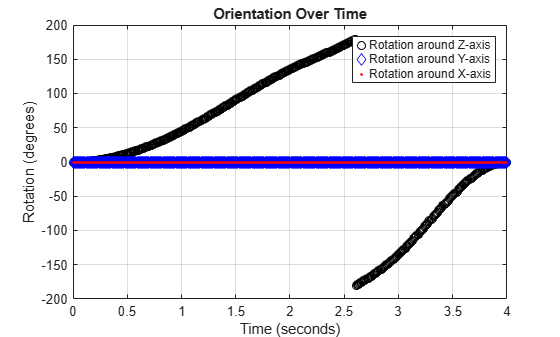The `waypointTrajectory` algorithm interpolates the waypoints to create a smooth trajectory. To return to the square trajectory, provide more waypoints, especially around sharp changes. To track corresponding times, waypoints, and orientation, specify all the trajectory info in a single matrix.

``` % Time, Waypoint, Orientation trajectoryInfo = [0, 0,0,0, 0,0,0; ... % Initial position 0.1, 0,0.1,0, 0,0,0; ... 0.9, 0,0.9,0, 0,0,0; ... 1, 0,1,0, 45,0,0; ... 1.1, 0.1,1,0, 90,0,0; ... 1.9, 0.9,1,0, 90,0,0; ... 2, 1,1,0, 135,0,0; ... 2.1, 1,0.9,0, 180,0,0; ... 2.9, 1,0.1,0, 180,0,0; ... 3, 1,0,0, 225,0,0; ... 3.1, 0.9,0,0, 270,0,0; ... 3.9, 0.1,0,0, 270,0,0; ... 4, 0,0,0, 270,0,0]; % Final position trajectory = waypointTrajectory(trajectoryInfo(:,2:4), ... TimeOfArrival=trajectoryInfo(:,1), ... Orientation=quaternion(trajectoryInfo(:,5:end),"eulerd","ZYX","frame"), ... SampleRate=100); ```

Create a figure and plot the initial position of the platform. In a loop, step through the trajectory to output the current position and current orientation. Plot the current position and log the orientation. Use `pause` to mimic real-time processing.

```figure(1) plot(waypoints(1,1),waypoints(1,2),"b*") title("Position") axis([-1,2,-1,2]) axis square xlabel("X") ylabel("Y") grid on hold on orientationLog = zeros(toa(end)*trajectory.SampleRate,1,"quaternion"); count = 1; while ~isDone(trajectory) [currentPosition,orientationLog(count)] = trajectory(); plot(currentPosition(1),currentPosition(2),"bo") pause(trajectory.SamplesPerFrame/trajectory.SampleRate) count = count+1; end hold off ```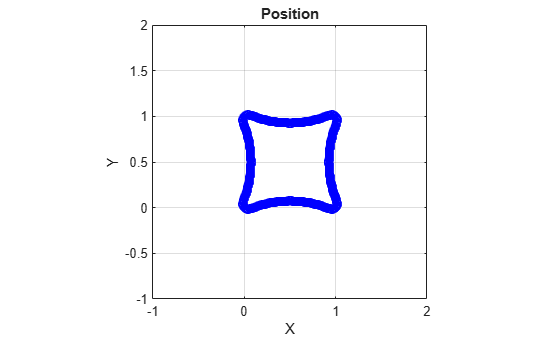The trajectory output now appears more square-like, especially around the vertices with waypoints.

Convert the orientation quaternions to Euler angles for easy interpretation, and then plot orientation over time.

```figure(2) eulerAngles = eulerd([orientation(1);orientationLog],"ZYX","frame"); t = 0:1/trajectory.SampleRate:4; eulerAngles = plot(t,eulerAngles(:,1),"ko", ... t,eulerAngles(:,2),"bd", ... t,eulerAngles(:,3),"r."); title("Orientation Over Time") legend("Rotation around Z-axis", ... "Rotation around Y-axis", ... "Rotation around X-axis", ... "Location", "SouthWest") xlabel("Time (seconds)") ylabel("Rotation (degrees)") grid on ```This example shows how to create an arc trajectory using the `waypointTrajectory` System object™. `waypointTrajectory` creates a path through specified waypoints that minimizes acceleration and angular velocity. After creating an arc trajectory, you restrict the trajectory to be within preset bounds.

Create an Arc Trajectory

Define a constraints matrix consisting of waypoints, times of arrival, and orientation for an arc trajectory. The generated trajectory passes through the waypoints at the specified times with the specified orientation. The `waypointTrajectory` System object requires orientation to be specified using quaternions or rotation matrices. Convert the Euler angles saved in the constraints matrix to quaternions when specifying the `Orientation` property.

``` % Arrival, Waypoints, Orientation constraints = [0, 20,20,0, 90,0,0; 3, 50,20,0, 90,0,0; 4, 58,15.5,0, 162,0,0; 5.5, 59.5,0,0 180,0,0]; trajectory = waypointTrajectory(constraints(:,2:4), ... TimeOfArrival=constraints(:,1), ... Orientation=quaternion(constraints(:,5:7),"eulerd","ZYX","frame")); ```

Call `waypointInfo` on `trajectory` to return a table of your specified constraints. The creation properties `Waypoints`, `TimeOfArrival`, and `Orientation` are variables of the table. The table is convenient for indexing while plotting.

```tInfo = waypointInfo(trajectory) ```
```tInfo = 4x3 table TimeOfArrival Waypoints Orientation _____________ ____________________ ________________ 0 20 20 0 {1x1 quaternion} 3 50 20 0 {1x1 quaternion} 4 58 15.5 0 {1x1 quaternion} 5.5 59.5 0 0 {1x1 quaternion} ```

The trajectory object outputs the current position, velocity, acceleration, and angular velocity at each call. Call `trajectory` in a loop and plot the position over time. Cache the other outputs.

```figure(1) plot(tInfo.Waypoints(1,1),tInfo.Waypoints(1,2),"b*") title("Position") axis([20,65,0,25]) xlabel("North") ylabel("East") grid on daspect([1 1 1]) hold on orient = zeros(tInfo.TimeOfArrival(end)*trajectory.SampleRate,1,"quaternion"); vel = zeros(tInfo.TimeOfArrival(end)*trajectory.SampleRate,3); acc = vel; angVel = vel; count = 1; while ~isDone(trajectory) [pos,orient(count),vel(count,:),acc(count,:),angVel(count,:)] = trajectory(); plot(pos(1),pos(2),"bo") pause(trajectory.SamplesPerFrame/trajectory.SampleRate) count = count + 1; end ```Inspect the orientation, velocity, acceleration, and angular velocity over time. The `waypointTrajectory` System object™ creates a path through the specified constraints that minimized acceleration and angular velocity.

```figure(2) timeVector = 0:(1/trajectory.SampleRate):tInfo.TimeOfArrival(end); eulerAngles = eulerd([tInfo.Orientation{1};orient],"ZYX","frame"); plot(timeVector,eulerAngles(:,1), ... timeVector,eulerAngles(:,2), ... timeVector,eulerAngles(:,3)); title("Orientation Over Time") legend("Rotation around Z-axis", ... "Rotation around Y-axis", ... "Rotation around X-axis", ... "Location","southwest") xlabel("Time (seconds)") ylabel("Rotation (degrees)") grid on figure(3) plot(timeVector(2:end),vel(:,1), ... timeVector(2:end),vel(:,2), ... timeVector(2:end),vel(:,3)); title("Velocity Over Time") legend("North","East","Down") xlabel("Time (seconds)") ylabel("Velocity (m/s)") grid on figure(4) plot(timeVector(2:end),acc(:,1), ... timeVector(2:end),acc(:,2), ... timeVector(2:end),acc(:,3)); title("Acceleration Over Time") legend("North","East","Down","Location","southwest") xlabel("Time (seconds)") ylabel("Acceleration (m/s^2)") grid on figure(5) plot(timeVector(2:end),angVel(:,1), ... timeVector(2:end),angVel(:,2), ... timeVector(2:end),angVel(:,3)); title("Angular Velocity Over Time") legend("North","East","Down") xlabel("Time (seconds)") ylabel("Angular Velocity (rad/s)") grid on ```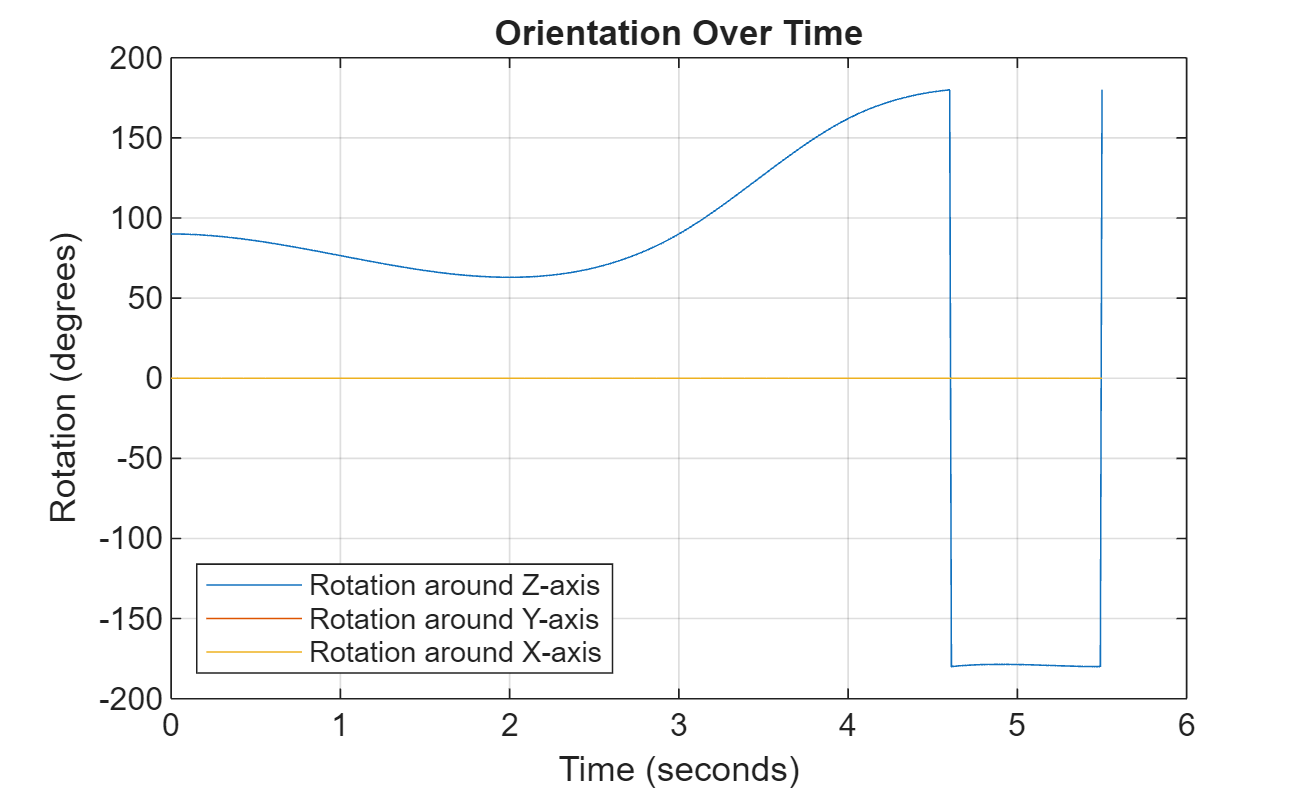Restrict Arc Trajectory Within Preset Bounds

You can specify additional waypoints to create trajectories within given bounds. Create upper and lower bounds for the arc trajectory.

```figure(1) xUpperBound = [(20:50)';50+10*sin(0:0.1:pi/2)';60*ones(11,1)]; yUpperBound = [20.5.*ones(31,1);10.5+10*cos(0:0.1:pi/2)';(10:-1:0)']; xLowerBound = [(20:49)';50+9*sin(0:0.1:pi/2)';59*ones(11,1)]; yLowerBound = [19.5.*ones(30,1);10.5+9*cos(0:0.1:pi/2)';(10:-1:0)']; plot(xUpperBound,yUpperBound,"r","LineWidth",2); plot(xLowerBound,yLowerBound,"r","LineWidth",2) ```To create a trajectory within the bounds, add additional waypoints. Create a new `waypointTrajectory` System object™, and then call it in a loop to plot the generated trajectory. Cache the orientation, velocity, acceleration, and angular velocity output from the `trajectory` object.

``` % Time, Waypoint, Orientation constraints = [0, 20,20,0, 90,0,0; 1.5, 35,20,0, 90,0,0; 2.5 45,20,0, 90,0,0; 3, 50,20,0, 90,0,0; 3.3, 53,19.5,0, 108,0,0; 3.6, 55.5,18.25,0, 126,0,0; 3.9, 57.5,16,0, 144,0,0; 4.2, 59,14,0, 162,0,0; 4.5, 59.5,10,0 180,0,0; 5, 59.5,5,0 180,0,0; 5.5, 59.5,0,0 180,0,0]; trajectory = waypointTrajectory(constraints(:,2:4), ... TimeOfArrival=constraints(:,1), ... Orientation=quaternion(constraints(:,5:7),"eulerd","ZYX","frame")); tInfo = waypointInfo(trajectory); figure(1) plot(tInfo.Waypoints(1,1),tInfo.Waypoints(1,2),"b*") count = 1; while ~isDone(trajectory) [pos,orient(count),vel(count,:),acc(count,:),angVel(count,:)] = trajectory(); plot(pos(1),pos(2),"gd") pause(trajectory.SamplesPerFrame/trajectory.SampleRate) count = count + 1; end ```The generated trajectory now fits within the specified boundaries. Visualize the orientation, velocity, acceleration, and angular velocity of the generated trajectory.

```figure(2) timeVector = 0:(1/trajectory.SampleRate):tInfo.TimeOfArrival(end); eulerAngles = eulerd(orient,"ZYX","frame"); plot(timeVector(2:end),eulerAngles(:,1), ... timeVector(2:end),eulerAngles(:,2), ... timeVector(2:end),eulerAngles(:,3)); title("Orientation Over Time") legend("Rotation around Z-axis", ... "Rotation around Y-axis", ... "Rotation around X-axis", ... "Location","southwest") xlabel("Time (seconds)") ylabel("Rotation (degrees)") grid on figure(3) plot(timeVector(2:end),vel(:,1), ... timeVector(2:end),vel(:,2), ... timeVector(2:end),vel(:,3)); title("Velocity Over Time") legend("North","East","Down") xlabel("Time (seconds)") ylabel("Velocity (m/s)") grid on figure(4) plot(timeVector(2:end),acc(:,1), ... timeVector(2:end),acc(:,2), ... timeVector(2:end),acc(:,3)); title("Acceleration Over Time") legend("North","East","Down") xlabel("Time (seconds)") ylabel("Acceleration (m/s^2)") grid on figure(5) plot(timeVector(2:end),angVel(:,1), ... timeVector(2:end),angVel(:,2), ... timeVector(2:end),angVel(:,3)); title("Angular Velocity Over Time") legend("North","East","Down") xlabel("Time (seconds)") ylabel("Angular Velocity (rad/s)") grid on ```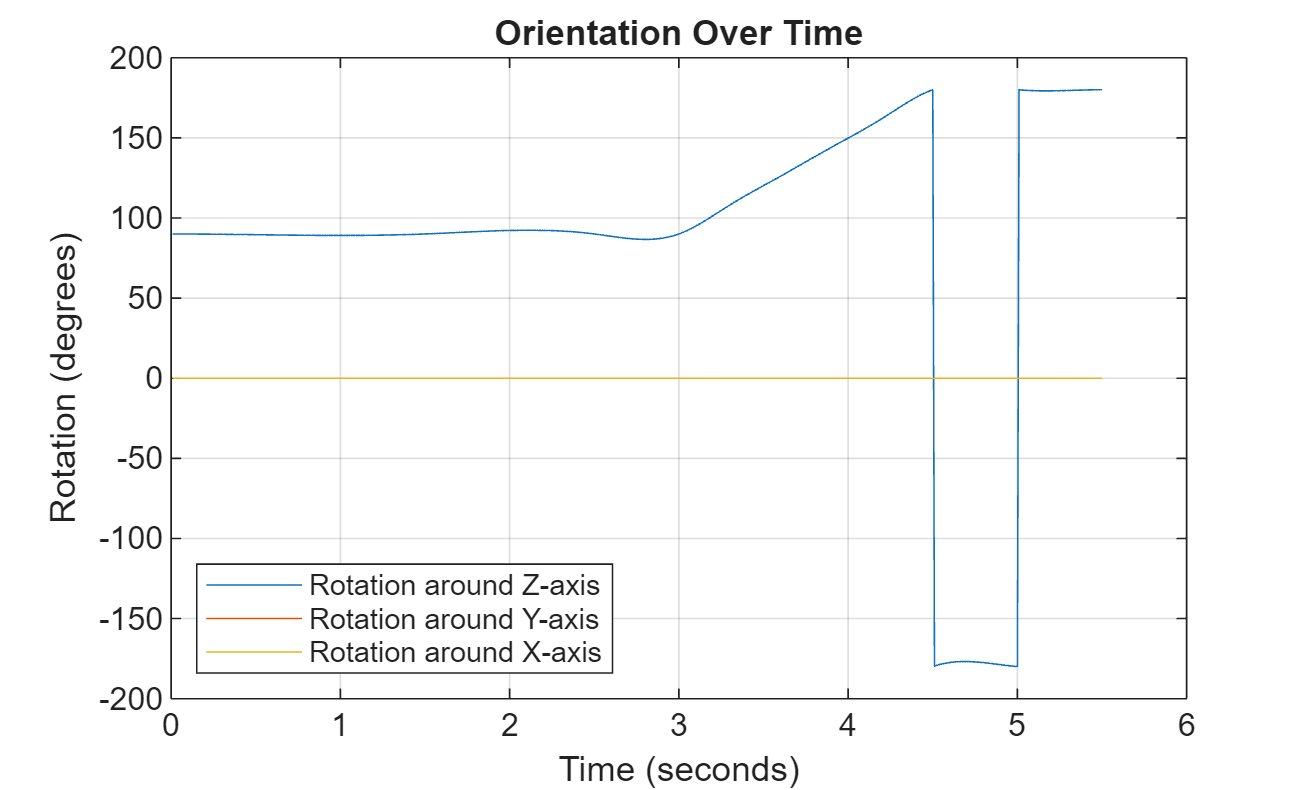Note that while the generated trajectory now fits within the spatial boundaries, the acceleration and angular velocity of the trajectory are somewhat erratic. This is due to over-specifying waypoints.

Consider a racetrack trajectory as the following.The four corner points of the trajectory are (0,0,0), (20,0,0), (20,5,0) and (0,5,0) in meters, respectively. Therefore, specify the waypoints of a loop as:

```wps = [0 0 0; 20 0 0; 20 5 0; 0 5 0; 0 0 0];```

Assume the trajectory has a constant speed of 2 m/s, and thus the velocities at the five waypoints are:

```vels = [2 0 0; 2 0 0; -2 0 0; -2 0 0; 2 0 0];```

The time of arrival for the five waypoints is:

`t = cumsum([0 20/2 5*pi/2/2 20/2 5*pi/2/2]');`

The orientation of the trajectory at the five waypoints are:

```eulerAngs = [0 0 0; 0 0 0; 180 0 0; 180 0 0; 0 0 0]; % Angles in degrees. % Convert Euler angles to quaternions. quats = quaternion(eulerAngs,"eulerd","ZYX","frame");```

Specify the sample rate as 100 for smoothing trajectory lines.

`fs = 100;`

Construct the `waypointTrajectory`.

```traj = waypointTrajectory(wps,SampleRate=fs, ... Velocities=vels,... TimeOfArrival=t,... Orientation=quats);```

Sample and plot the trajectory.

```[pos, orient, vel, acc, angvel] = traj(); i = 1; spf = traj.SamplesPerFrame; while ~isDone(traj) idx = (i+1):(i+spf); [pos(idx,:), orient(idx,:), ... vel(idx,:), acc(idx,:), angvel(idx,:)] = traj(); i = i+spf; end ```

Plot the trajectory and the specified waypoints.

```plot(pos(:,1),pos(:,2), wps(:,1),wps(:,2), "--o") xlabel("X (m)") ylabel("Y (m)") zlabel("Z (m)") legend({"Trajectory", "Waypoints"}) axis equal```Create a `waypointTrajectory` object that connects two waypoints. The velocity of the trajectory at the two waypoints is 0 $\mathrm{m}/\mathrm{s}$ and 10 $\mathrm{m}/\mathrm{s}$, respectively. Restrict the jerk limit to 0.5 $\mathrm{m}/{\mathrm{s}}^{3}$ to enable the trapezoidal acceleration profile.

```waypoints = [0 0 0; 10 50 10]; speeds = [0 10]; jerkLimit = 0.5; trajectory = waypointTrajectory(waypoints,GroundSpeed=speeds,JerkLimit=jerkLimit);```

Obtain the initial time and final time of the trajectory by querying the `TimeOfArrival` property. Create time stamps to sample the trajectory.

```t0 = trajectory.TimeOfArrival(1); tf = trajectory.TimeOfArrival(end); sampleTimes = linspace(t0,tf,100);```

Obtain the position, velocity, and acceleration information at these sampled time stamps using the `lookupPose` object function.

`[position,~,velocity,acceleration,~] = lookupPose(trajectory,sampleTimes);`

Plot the trajectory.

```figure() plot3(position(:,1),position(:,2),position(:,3)) xlabel("x (m)") ylabel("y (m)") zlabel("z (m)") title("Trajectory")```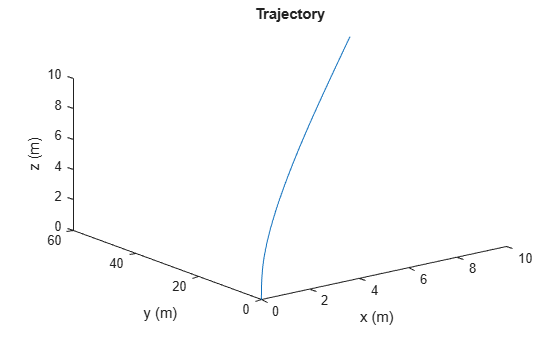Plot the velocity profile.

```figure() subplot(3,1,1) plot(sampleTimes,velocity(:,1)); ylabel("v_x (m/s)") title("Velocity Profile") subplot(3,1,2) plot(sampleTimes,velocity(:,2)); ylabel("v_y (m/s)") subplot(3,1,3) plot(sampleTimes,velocity(:,3)); ylabel("v_z (m/s)") xlabel("Time (sec)")```Plot the acceleration profile. From the results, the acceleration profile of the planar motion is trapezoidal.

```figure() subplot(3,1,1) plot(sampleTimes,acceleration(:,1)); axis padded ylabel("a_x (m/s^2)") title("Acceleration Profile") subplot(3,1,2) plot(sampleTimes,acceleration(:,2)); ylabel("a_y (m/s^2)") axis padded subplot(3,1,3) plot(sampleTimes,acceleration(:,3)); ylabel("a_z (m/s^2)") xlabel("Time (sec)")```## Algorithms

The `waypointTrajectory` System object defines a trajectory that smoothly passes through waypoints. The trajectory connects the waypoints through an interpolation that assumes the gravity direction expressed in the trajectory reference frame is constant. Generally, you can use `waypointTrajectory` to model platform or vehicle trajectories within a hundreds of kilometers distance span.

The planar path of the trajectory (the x-y plane projection) consists of piecewise, clothoid curves. The curvature of the curve between two consecutive waypoints varies linearly with the curve length between them. The tangent direction of the path at each waypoint is chosen to minimize discontinuities in the curvature, unless the course is specified explicitly via the `Course` property or implicitly via the `Velocities` property. Once the path is established, the object uses cubic Hermite interpolation to compute the location of the vehicle throughout the path as a function of time and the planar distance traveled. If the `JerkLimit` property is specified, the objects produces a horizontal trapezoidal acceleration profile for any segment that is between two waypoints. The trapezoidal acceleration profile consists of three subsegments:

• A constant-magnitude jerk subsegment

• A constant-magnitude acceleration subsegment

• A constant-magnitude jerk subsegment

The normal component (z-component) of the trajectory is subsequently chosen to satisfy a shape-preserving piecewise spline (PCHIP) unless the climb rate is specified explicitly via the `ClimbRate` property or the third column of the `Velocities` property. Choose the sign of the climb rate based on the selected `ReferenceFrame`:

• When an 'ENU' reference frame is selected, specifying a positive climb rate results in an increasing value of z.

• When an 'NED' reference frame is selected, specifying a positive climb rate results in a decreasing value of z.

You can define the orientation of the vehicle through the path in two primary ways:

• If the `Orientation` property is specified, then the object uses a piecewise-cubic, quaternion spline to compute the orientation along the path as a function of time.

• If the `Orientation` property is not specified, then the yaw of the vehicle is always aligned with the path. The roll and pitch are then governed by the `AutoBank` and `AutoPitch` property values, respectively.

`AutoBank``AutoPitch`Description
`false``false`The vehicle is always level (zero pitch and roll). This is typically used for large marine vessels.
`false``true`The vehicle pitch is aligned with the path, and its roll is always zero. This is typically used for ground vehicles.
`true``false`The vehicle pitch and roll are chosen so that its local z-axis is aligned with the net acceleration (including gravity). This is typically used for rotary-wing craft.
`true``true`The vehicle roll is chosen so that its local transverse plane aligns with the net acceleration (including gravity). The vehicle pitch is aligned with the path. This is typically used for two-wheeled vehicles and fixed-wing aircraft.

## Version History

Introduced in R2018b

expand all